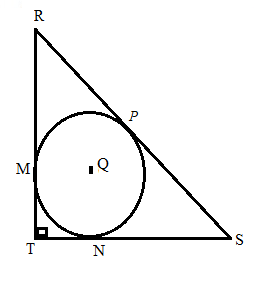Chapter 6.3, Problem 36E### Elementary Geometry for College St...

6th Edition
Daniel C. Alexander + 1 other
ISBN: 9781285195698

#### Solutions

Chapter
Section### Elementary Geometry for College St...

6th Edition
Daniel C. Alexander + 1 other
ISBN: 9781285195698
Textbook Problem
1 views

# Given: ⊙ Q is inscribed in isosceles right Δ R S T . The perimeter of Δ R S T is 8 + 4 2 Find: TMTo determine

To find:

To find TM.

Explanation

Given that, Q is inscribed in isosceles right ΔRST. The perimeter of ΔRST is 8+42.

The tangents segments from an external point are congruent. Therefore,

RP=RM=xSP=SN=yTN=TM=z

The diagrammatic representation is given below,

Perimeter of the ΔRST is 8+42.

That is RT+TS+RS=8+42

Given that ΔRST is an isosceles triangle. Therefore, RT=TS.

Then the above equation become the following,

RT+TS+RS=8+42RT+RT+RS=8+422RT+RS=8+42.........(1)

By using the Pythagorean theorem to get the following,

RT2+TS2=RS2RT2+RT2=RS22RT2=RS2. Since RT=TS

Taking square root on both sides to get the following,

RS=RT2...........(2)

Substitute equation (2) in (1) to get the following,

2RT+RT2=8+42RT(2+2)=8+42RT=4(2+2)(2+2)RT=4

Also, know that RT=TS=4

By using the Pythagorean theorem to get the following,

RT2+TS2=RS242+42=RS216+16=RS2RS=32RS=42.

The value of RT=4,TS=4,andRS=42

We know that,

RS=RP+PS=x+yTS=TN+NS=z+yRT=RM+MT=x+z

Substitute the value of RT=4,TS=4,andRS=42 in the above equation to get the following,

x+y=42

### Still sussing out bartleby?

Check out a sample textbook solution.

See a sample solution

#### The Solution to Your Study Problems

Bartleby provides explanations to thousands of textbook problems written by our experts, many with advanced degrees!

Get Started

#### Expand each expression in Exercises 122. (4y2)y

Finite Mathematics and Applied Calculus (MindTap Course List)

#### Area In Exercises 73-76, find the area or the given region. y=3x+2x2+9

Calculus: Early Transcendental Functions (MindTap Course List)

#### Using a binomial series, the Maclaurin series for is:

Study Guide for Stewart's Multivariable Calculus, 8th